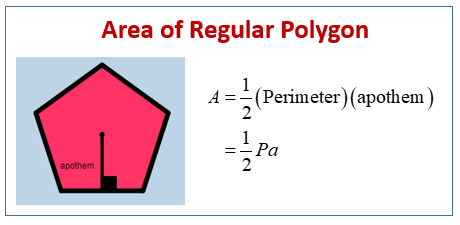# Math CSET II: How to Find the Area of a Regular Polygon

First of all let’s get this out of the way, the CSET will never ask you directly to do anything.  Part of the complication of this test is trying to figure out what you need to do AND knowing the skills to do so.  A staple question on the MATH CSET II is finding area of a regular polygon whether directly or you had to answer the riddle from a troll to be let into the testing center.

## What is a Regular Polygon?

A regular polygon is a closed shape such that every side is the SAME length.  We denote the number of sides with the letter n.  For example:## How Do I Find The Area?

Since this post is designed to get straight to the point here is the formulas.  They all have wonderful proofs, but that’s not why we’re here.

If you know the number of sides n and the side length s:If you know the perimeter and the apothem:

What the hell is an apothem?  Its the line perpendicular from the center to any side.  For example:The formula for area if you know the apothem a and the perimeter p is:These are the two main ones.  Since you are allowed a graphing calculator for the Math CSET 2 you can just plug all these in and hit enter.  Make sure you round correctly 🙂

As always share the post if you found it helpful.  The more math teachers we have the better our world becomes.